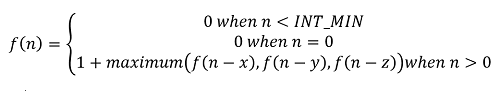# Maximize the cut segments

Maximize the cut segments: It is a classic dynamic programing problem which has been featured in interview rounds of amazon.
Submitted by Radib Kar, on April 10, 2020

Description:

In this article we are going to review classic dynamic programing problem which has been featured in interview rounds of amazon.

Problem statement:

Given an integer N denoting the Length of a rod, one needs to cut the rod in such a way that the cut length of the rod segment each time is integer either x, y or z and after performing all cutting operation the total number of cut segments must be maximum. You need to find the maximum number of segments possible to cut.

Example:

```    Input1
Rod length: 6
Segment's length:
x=3,y=2,z=1

Output1
Maximum number of cut segments: 6

Input2
Rod length: 5
Segment's length:
x=5,y= 3,z= 2

Output2
Maximum number of cut segments: 2
```

Example with explanation:

```    For the first example,
Length of the rod is: 4
Three cut segments’ length is 3, 2, 1 respectively.
To get maximum cuts we would cut in segments of length
1 which will have outcome 6.

For the second example,
Length of the rod is: 5
Three cut segments’ length is 5, 3, 2 respectively.
To get maximum cuts we would cut in segments of
length 2 & length 3 which will have outcome 2.
```

Solution Approach:

This problem can be solved recursively.

```    f(n) = number of cut segments for rod with length n
f(n) = 1+maximum(f(n-x),f(n-y),f(n-z))
```

With base case,

```    f(negative number) = INT_MIN
f(0) = 0
```

So, the recursive function definition would be:Solving the above recursive function would give the result.

The function is defined below,

```    Function cutTheRod(n):
If n<0
return INT_MIN
If n==0
return 0;
return 1+maximum(f(n-x),f(n-y),f(n-z));
End Function
```

If you draw any recursion tree, you will find many overlapping subproblems and hence we need to store them and use dynamic Programing to solve.

Before going to a dynamic programming solution, let’s talk about one observation. The thing is as we need to find the maximum number of cuts, we would like to use the minimum long segment as much as possible. It seems that greedy would have worked by choosing minimum long segments and then upgrading to other segments. If greedy works for a test case, the solution is guaranteed to be optimal. Earlier we have seen that greedy may not be optimal, but it’s not in this case. In this case greedy is optimal. The issue is greedy doesn’t work for all test cases. Think of a test case such that rod length is 9:

And segment lengths are: 5, 3, 2

Greedy would have cut 4 segments of length 4 and then fails eventually as no segment of length 1. That’s why we need DP, but there is one greedy technique that can be added along to optimize. Since, we need to find maximum cuts, find out the minimum segment and check whether the rod length is divisible by the minimum length or not. If it’s divisible that ensures that rod length/minimum cut length is the maximum number of cut segments. You can check for example 1 there are 4 cut segments, which is rod length(4)/ minimum segment length(1) since rod length was divisible by minimum segment length.

The Dynamic programing solution can be found below:

1. Initialize dp[n+1] in the following way.
```    dp=0
for  i=1 to n
dp[i]=INT_MIN
```
2. Fill the table
```    for i=1 to i++)
if(i-x>=0 && dp[i]<1+dp[i-x])
dp[i]=1+dp[i-x];

if(i-y>=0 && dp[i]<1+dp[i-y])
dp[i]=1+dp[i-y];

if(i-z>=0 && dp[i]<1+dp[i-z])
dp[i]=1+dp[i-z];
end for
```
3. ```    If dp[n]<=0
Cutting not possible
Else
That's the result.
```

C++ Implementation:

```#include <bits/stdc++.h>
using namespace std;

int cuttingrod(int n, int x, int y, int z)
{
int minimum;
if (x < y && x < z)
minimum = x;
if (y < x && y < z)
minimum = y;
if (z < y && z < x)
minimum = z;

if (minimum != 0 && n % minimum == 0)
return (n / minimum);

int dp[n + 1];
for (int i = 1; i <= n; i++)
dp[i] = INT_MIN;
dp = 0;
for (int i = 1; i <= n; i++) {

if (i - x >= 0 && dp[i] < 1 + dp[i - x]) {
dp[i] = 1 + dp[i - x];
}
if (i - y >= 0 && dp[i] < 1 + dp[i - y]) {
dp[i] = 1 + dp[i - y];
}
if (i - z >= 0 && dp[i] < 1 + dp[i - z]) {
dp[i] = 1 + dp[i - z];
}
}
return dp[n];
}

int main()
{
int t, n, x, y, z;

cout << "Original rod length:\n";
cin >> n;
cout << "First cut segment length,x:\n";
cin >> x;
cout << "Second cut segment length,y:\n";
cin >> y;
cout << "Third cut segment length,z:\n";
cin >> z;

int ans = cuttingrod(n, x, y, z);
if (ans > 0)
cout << "Max number of cut segments: " << ans << endl;
else
cout << "Can't be cut down\n";

return 0;
}
```

Output

```RUN 1:
Original rod length:
6
First cut segment length,x:
3 2 1
Second cut segment length,y:
Third cut segment length,z:
Max number of cut segments: 6

RUN 2:
Original rod length:
17
First cut segment length,x:
11
Second cut segment length,y:
13
Third cut segment length,z:
9
Can't be cut down
```